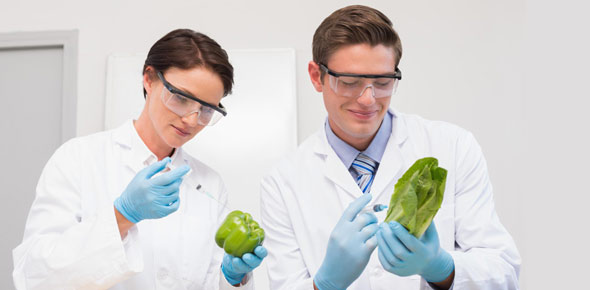# Food Science Midterm Examination

100 Questions | Attempts: 96
ShareSettingsThis Examination covers the following Standards: FCS-FS-1. Students will define food science and explore careers in food science.   ; a) Define the study of food science and summarize how food products and processing methods have changed in modern history due to contributions of food scientists.   ; b) Describe the history of the development of food and food systems emphasizing the transition from hunting and gathering to farming and then to market-based societies. &n

• 1.
The energy a substance uses or releases to change phase.
• A.

Latent heat

• B.

Heat of vaporization

• C.

Sublimation

• D.

Electronegativity

• 2.
The MOST reliable way to find a pH level is with _______________.
• A.

Litmus paper

• B.

Anthocyanins

• C.

A pH scale

• D.

A ph meter

• 3.
Blood is kept at a healthy, slightly basic pH by ___________.
• A.

An adequate supply of basic foods in the diet

• B.

Basic fluids secreted in the digestive tract

• C.

Various buffering agents in the blood

• D.

Antacid dietary supplements

• 4.
On the pH scale, ___________________.
• A.

Higher values indicate greater acidity

• B.

Lower values indicate greater acidity

• C.

A neutral solution has a value of 14

• D.

None of the above

• 5.
A base in which few molecules ionize is called _______________.
• A.

Weak

• B.

Dilute

• C.

Neutral

• D.

All of the above

• 6.
One sign that neutralization has occurred is _______________.
• A.

A blue indicator

• B.

A slippery feel

• C.

The presence of water

• D.

The presence of anthocyanins

• 7.
Acids in foods pose little health risk because __________________________.
• A.

They are only slightly soluble

• B.

They are weak

• C.

They are not often used in food

• D.

All of the above

• 8.
Hydrogen ions and hydroxide ions ________________.
• A.

Carry opposite charges

• B.

Are products of ionization

• C.

May combine to form water

• D.

All are true

• 9.
Five liters of a 3 M solution of ammonia contains _________________.
• A.

0.66 moles of ammonia

• B.

1.66 moles of ammonia

• C.

8 moles of ammonia

• D.

15 moles of ammonia

• 10.
A base in solution ________________.
• A.

Produces hydroxide ions

• B.

Produces hydrogen ions

• C.

Neutralizes the solution

• D.

Lower's the solution's pH

• 11.
To do a titration of an acid solution, you need to know _________________.
• A.

The volume of the acid solution

• B.

The volume of a basic solution

• C.

The concentration of the basic solution

• D.

All are true

• 12.
Having an equal number of hydrogen atoms and hydrogen ions.
• A.

Neutral

• B.

Molarity

• C.

Base

• D.

Acid

• 13.
A stage of titration that indicates neutralization
• A.

Equivalence point

• B.

Hydrogen ion

• C.

Water

• D.

Indicator

• 14.
A procedure done to determine the molarity of a solution
• A.

Titration

• B.

Molarity

• C.

Concentration

• D.

Tonsilectomy

• 15.
A measure of relative acidity in a solution
• A.

PH

• B.

Titration

• C.

Buffer

• D.

Base

• 16.
A substance that releases hydrogen ions in a solution
• A.

Acid

• B.

Base

• C.

Neutral

• D.

Buffer

• 17.
A positively charged particle
• A.

Hydrogen ion

• B.

Hydroxide ion

• C.

Titration

• D.

Anthocyanin

• 18.
A substance that responds to pH by changing color
• A.

Indicator

• B.

PH

• C.

Water

• D.

Base

• 19.
A measurement that tells the amount of a substance per unit of volume
• A.

Concentration

• B.

Molarity

• C.

Titration

• D.

Indicator

• 20.
A result of a hydrogen ion and hydroxide ion bonding
• A.

Water

• B.

Base

• C.

Neutral

• D.

Acid

• 21.
A substance that releases hydroxide ions in solution
• A.

Base

• B.

Neutral

• C.

Acid

• D.

Molarity

• 22.
A measurement that indicates the moles of solute per liter of solution
• A.

Molarity

• B.

Polarity

• C.

Equivalence point

• D.

Anthocyanin

• 23.
A substance that prevents blood from becoming too acidic or basic
• A.

Buffer

• B.

Base

• C.

Acid

• D.

PH

• 24.
A chemical reaction that produces water and salts
• A.

Neutralization

• B.

Aerobic activity

• C.

Anaerobic activity

• D.

Concentration

• 25.
A plant pigment used to test pH
• A.

Anthocyanin

• B.

Acid

• C.

Buffer

• D.

Titration

## Related TopicsBack to top
×

Wait!
Here's an interesting quiz for you.